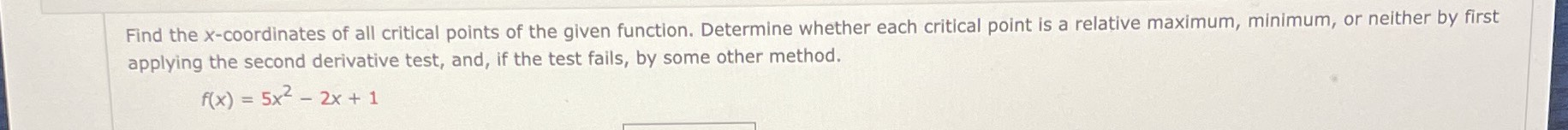### Still have math questions?

Algebra
QuestionFind the $$x$$ -coordinates of all critical points of the given function. Determine whether each critical point is a relative maximum, minimum, or neither by first applying the second derivative test, and, if the test fails, by some other method.

$$f ( x ) = 5 x ^ { 2 } - 2 x + 1$$

critical point is $$(\frac{1}{5}, \frac{4}{5})$$